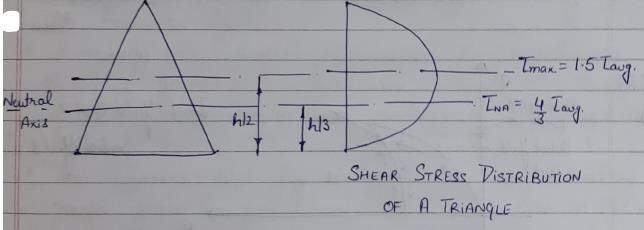Courses

# Test: Bending & Shear Stress - 1

## 10 Questions MCQ Test Solid Mechanics | Test: Bending & Shear Stress - 1

Description
This mock test of Test: Bending & Shear Stress - 1 for Civil Engineering (CE) helps you for every Civil Engineering (CE) entrance exam. This contains 10 Multiple Choice Questions for Civil Engineering (CE) Test: Bending & Shear Stress - 1 (mcq) to study with solutions a complete question bank. The solved questions answers in this Test: Bending & Shear Stress - 1 quiz give you a good mix of easy questions and tough questions. Civil Engineering (CE) students definitely take this Test: Bending & Shear Stress - 1 exercise for a better result in the exam. You can find other Test: Bending & Shear Stress - 1 extra questions, long questions & short questions for Civil Engineering (CE) on EduRev as well by searching above.
QUESTION: 1

### A simply supported beam of span L carries a concentrated load W at its mid-span. If the width b of the beam is constant and its depth is varying throughout the span, then what should be its mid-span depth, when design stress is f?

Solution:

Let mid span depth is d. Then BM at a distance L/2 from one end.
∴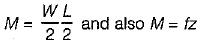∴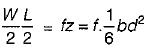∴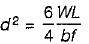or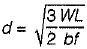QUESTION: 2

### A beam of T-section has I = 1200 cm4 and depth = 12 cm. Flange of the section is in compression. If the maximum tensile stress is two times the maximum compressive stress, what is section modulus in compression?

Solution: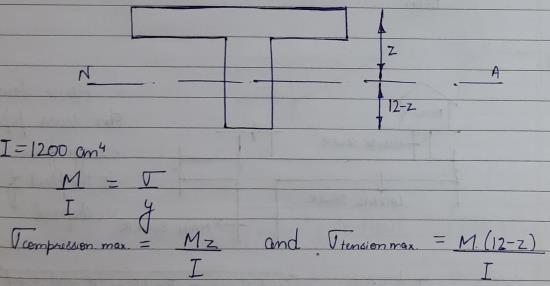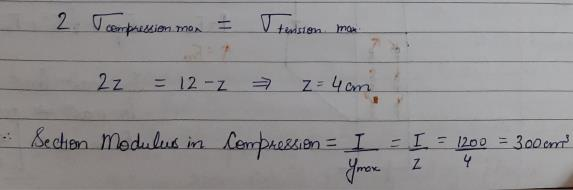QUESTION: 3

### The width of a beam of uniform strength having a constant depth d, length L, simply supported at the ends with a central load W is

Solution:

As we know,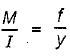∴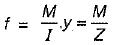Now,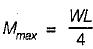∴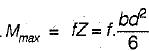⇒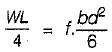⇒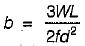QUESTION: 4

A beam of rectangular cross-section is 100 mm wide and 200 mm deep. If the section is subjected to a shear force of 20 kN, the maximum shear stress is

Solution:

The maximum shear stress in a rectangular beam occurs at NA and is given by,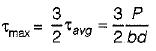∴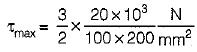= 1.5 N/mm2

QUESTION: 5

The ratio of the flexural strengths of two square beams one placed with two sides horizontal and the other placed with one diagonal vertical and other horizontal is

Solution: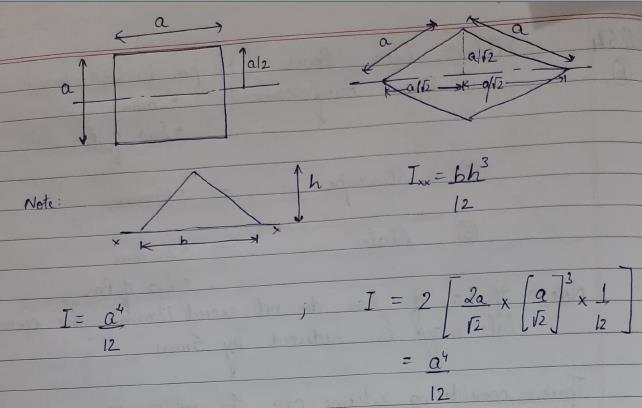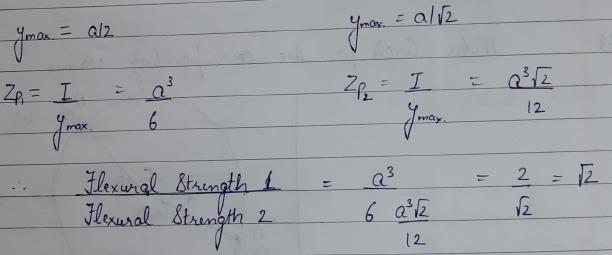QUESTION: 6

The modular ratio of the materials used in the flitched beam is 10 and the ratio of the allowable stresses is also 10. Four different sections of the beam are: shown in the given figures. The material shown hatched has larger modulus of elasticity and allowable stress than the rest.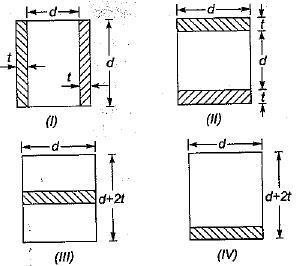Which one of the following statements is true for the beam under consideration ?

Solution:

In the flitched beams there are two principles to be followed in analysis,
(i) strain diagram is linear
(ii) The radius of curvature of both materials is same at all the points.

• Allowable stress in weak material = f
• Allowable stress in strong material = 10 f
• Modulus of elasticity of weak material = E
• Modulus of elasticity of strong material = 10E
• Maximum strain in weak material = f/E
• Maximum strain in strong material =10f/10E = f/E
• The hatched material can be replaced by equivalent weak material when its width is multiplied by modular ratio. So the equivalent sections are: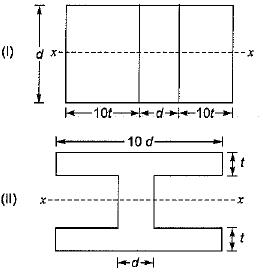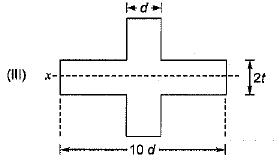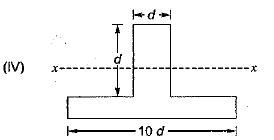It is very clear that section modulus of section-ll is more than that of section-Ill as more area is close to the neutral axis in section-lI. Section-IV is unequal and its section modulus in tension and compression will be different. Since the strain is (f/E) at topmost and at bottom most fibre it is less than f/E.
So its capacity will be less than that of section-ll. Comparing section-l and section-ll, more area is away from the neutral axis in section-ll, so the moment capacity of section-ll is more than that of section-l. Thus section-ll has higher capacity.

QUESTION: 7

Cross-sections of two beams A (600 mm x 200mm) and B (800 mm x 60 mm) are shown in the figure given above. Both the beams have the same material. By how many times is the beam A stronger than the beam B in resisting bending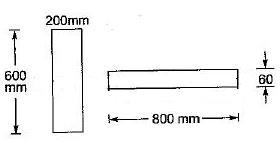Solution: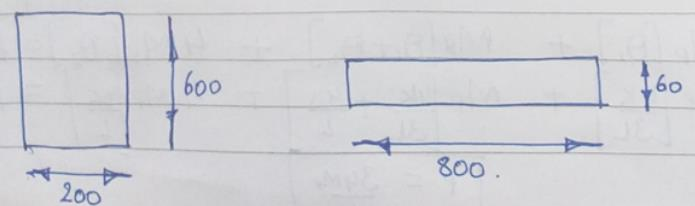Z = I/(y(max)) = bd2 /6
Za = (200) (600)2 / 6
Zb = (800) (600)2/6
Za / Zb = 25

QUESTION: 8

A beam of uniform strength refers to which one of the following?

Solution:
• Beam with constant moment of inertia will be prismatic beam.
• Beam in which bending stress is uniform at maximum bending moment cross-section will form a plastic hinge at that section..
• Beam of uniform strength are of two types,
(i) Beams of constant width
(ii) Beams of constant depth
QUESTION: 9

Maximum shear stress in a circular cross-section is

Solution:
QUESTION: 10

Shear stress in a beam having triangular cross-section is maximum at

Solution: